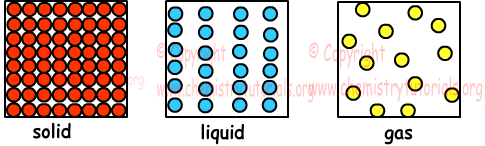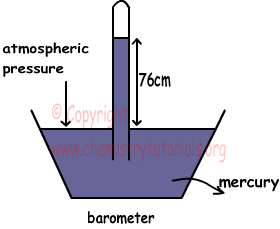EN | ES | DE | NL | RU

## Gases with Examples

Gases with Examples

Gas is one of the phases of matter. Distances between atoms or molecules in gas phase are larger than solids and liquids. Because of this reason, we can compress gases. Gases do not have specific volumes, they fill the container. Gases also have property of diffusing. We will explain four basic concepts helping us in examining gases, moles, volume, temperature and pressure. Picture given below show differences between structures of solid, liquid and gas.Mole (n)

In last unit we learn mole concept. 1 mole gas contains 6,02x1023 atoms or molecules. For example, 16 g O and 16 g N has different volumes, on the contrary 16 g O and 14 g N  has same volume since their number of atoms and moles are equal. We use following formulas to find mole of gas.

n=m/M

where; n is number of mole, m is mass, M is molar mass of element or compound.

OR;

n=N/NA

where, n is number of mole, N is number of atoms or molecules, NA is Avogadro's Number.

Volume (V)

Volume of gas is equal to volume of container. They have no specific volumes. Under standard temperature and pressure 1 mole gas has 22,4 liters volume. Units of volume we use here is liter.

Temperature (T)

In calculations of gases absolute temperature (K) is used. In -273, diffusion of gases is zero and in nature Kelvin is the unit of temperature and relation between K and 0C is;

T(K)=t(0C)+273

Example: Find value of 1200C in terms of Kelvin.

T=120+273=393 K

Example: Find value of 300 K in terms of 0C.

T=t+273

300=t+273

t=27 0C

Pressure:

Pressure is the force acting perpendicularly on unit surface. Unit of pressure is mm Hg, cm Hg or atm. In general, (atmospheric pressure) atm is used. Reason of the gas pressure is motion and collision of gas particles on surfaces. In measuring gas pressure, there are two methods we should learn, measuring pressure of gas and atmospheric pressure. Picture given below shows a method for measuring atmospheric pressure.This setup is a simple barometer that helps us to measure atmospheric pressure. In this system, empty tube is immersed into the tank filled with mercury. After this step some of the mercury rises in the tube at level 76cm. In liquids, pressures are equal at points in same level. Thus, atmospheric pressure on surface of liquid must be balanced by pressure of mercury in the tube. 76 cm shows us, this amount of mercury pressure balance atmospheric pressure. Let P0 is the atmospheric pressure then;
P0=h cm Hg

P0=76 cm Hg at sea level.

h depends on;

density of the liquid put into container

h does not depend on cross section area of tube.

Gases Exams and  Problem Solutions

The Original Author: